# 2 Dimensional Motion

Fall 2008 Rick Mills Art 130 Introduction to Glass at UH Manoa
These are the projects that I did.

Preface: This tutorial explains motion in 2 dimensions. I assume you know that "Vi" is initial velocity, "Vf" is final velocity, "-delta-X" is change in X, a is acceleration, and "t" is time... I also assume prior knowledge of algebra. This may be comprehensive but I'm not gonna teach you algebra, or how to ride elephants... just 2 dimensional motion.

Ok lets assume that we put your sister in a wagon. (if you dont have a sister you cant do physics)If we just roll her around that is only in one dimension. so...

Lets put the wagon on a catapult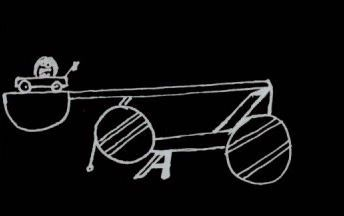Now we are going to launch her at 92 meters / second at 30 degrees above the horizontal. (yes that is about a foot ball field a second)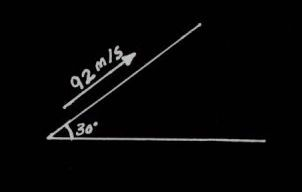Now what we want to know is where to put the comicly small pool of water for her to land in.(-deltaX- (not a spy))

I suggest you first write down the "Big 3" equations.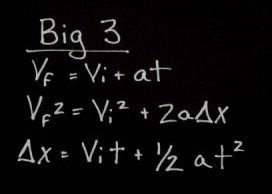I also write out a table I will fill in for all my motion problems (not gyrating or kicking people, just for physics)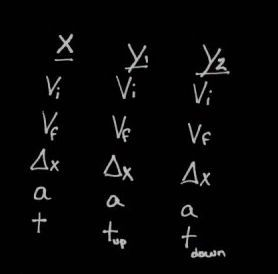Now we know that we are looking for how far your sister will fly (thats right your sister IS fly ;) ). To do this we are going to need to use the big 3 equations. We can not just find how far she flys because to find how far she goes we need to know how fast she is going in a horizontal direction, and for how long.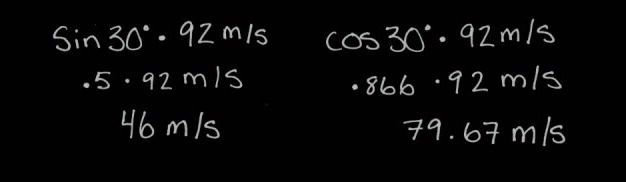The sin of the angle times the speed of the hypotonuse (the angled direction) will tell us how fast she is flying up, cos of the angle multiplied by the hypotonuse will give us her horizontal speed. Now we can fill in our table with what we know.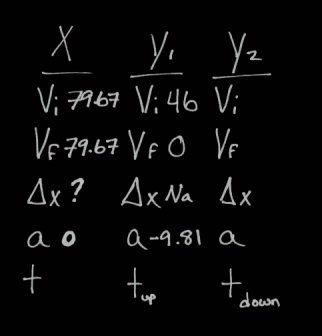Because we do not know the amount of time your sister will be flying along the horizontal we need to find the time. Because of magic the amount of time she will be flying horizontally is the same as the amount of time she is flying both up and down. And since we have enough information to find how long she is flying up and down ... we do so (because we CAN!)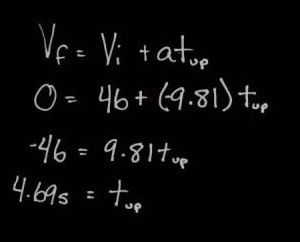This shows how long it takes her to reach the apex of he flight up. Again because of magic we know that it will take the same amount of time for her to come down (yes magic). So now we can fill in the rest of our table for the motion in the X ;)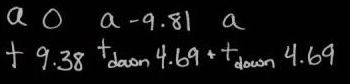Now we can calculate how far she will fly using a "Big 3" equation.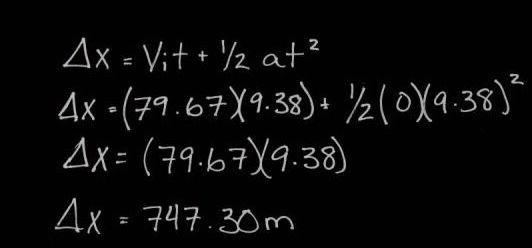And thus your sister will fly 747.30 meters.

Special thanks to: Your sister ;)